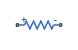# Resistor

Linear resistor in electrical systems

•Libraries:
Simscape / Foundation Library / Electrical / Electrical Elements

## Description

The Resistor block models a linear resistor, described with the following equation:

`$V=I·R$`

where:

• V is voltage.

• I is current.

• R is resistance.

Connections + and – are conserving electrical ports corresponding to the positive and negative terminals of the resistor, respectively. By convention, the voltage across the resistor is given by V(+) – V(–), and the sign of the current is positive when flowing through the device from the positive to the negative terminal. This convention ensures that the power absorbed by a resistor is always positive.

### Variables

To set the priority and initial target values for the block variables prior to simulation, use the Initial Targets section in the block dialog box or Property Inspector. For more information, see Set Priority and Initial Target for Block Variables.

Nominal values provide a way to specify the expected magnitude of a variable in a model. Using system scaling based on nominal values increases the simulation robustness. Nominal values can come from different sources, one of which is the Nominal Values section in the block dialog box or Property Inspector. For more information, see Modify Nominal Values for a Block Variable.

## Ports

### Conserving

expand all

Electrical conserving port associated with the resistor positive terminal.

Electrical conserving port associated with the resistor negative terminal.

## Parameters

expand all

Resistance value.

## Version History

Introduced in R2007a